Jungbrunnen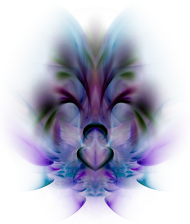3,00 €
AQUAM 2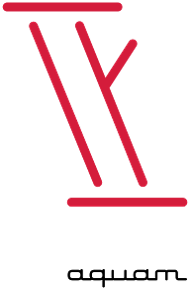3,00 €
Symbol AQUAM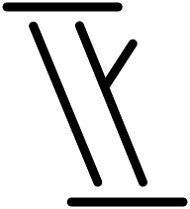4,00 €
SQUARE DANCE - LOVE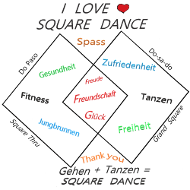9,00 €
Ich sehe keinen Grund, mich an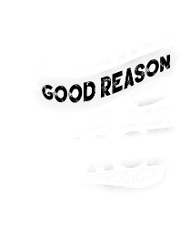8,46 €
Gärtner beste Therapie Tomate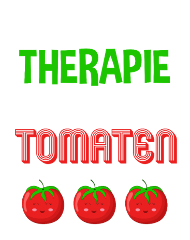7,50 €
original since 1966 simply the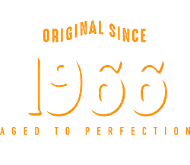3,50 €
original since 1986 simply the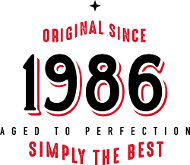3,50 €
Gesund dank Hund - Spruch Hund4,48 €
Gesund dank Hund - Spruch Hund4,48 €
original since 1976 simply the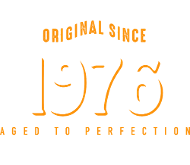3,50 €
original since 1966 simply the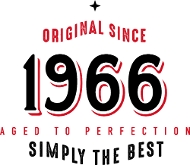3,50 €
original since 1976 simply the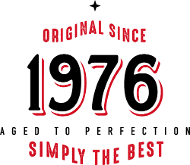3,50 €
original since 1986 simply the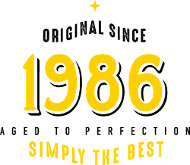3,50 €
original since 1976 simply the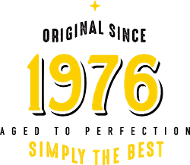3,50 €
original since 1986 simply the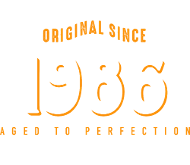3,50 €
original since 1966 simply the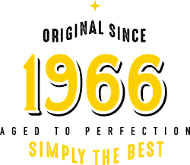3,50 €
original since 1948 simply the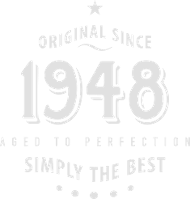3,00 €
original since 1988 simply the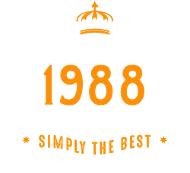3,00 €
original since 1978 simply the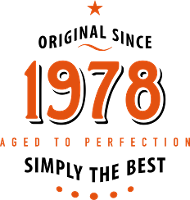3,00 €
original since 1948 simply the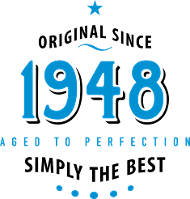3,00 €
original since 1968 simply the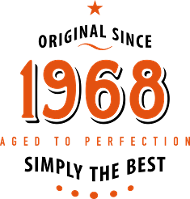3,00 €
original since 1978 simply the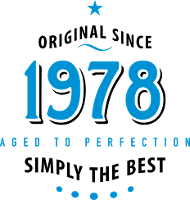3,50 €
original since 1978 simply the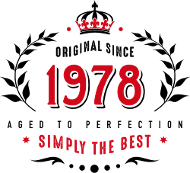3,50 €
original since 1968 simply the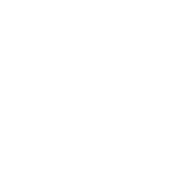3,50 €
original since 1967 simply the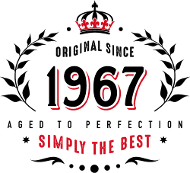3,50 €
original since 1948 simply the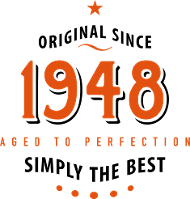3,50 €
original since 1988 simply the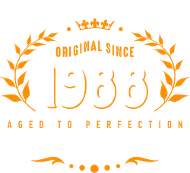3,50 €
original since 1948 simply the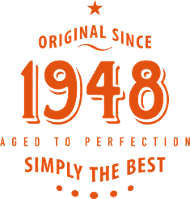3,00 €
original since 1958 simply the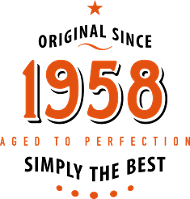3,50 €
original since 1958 simply the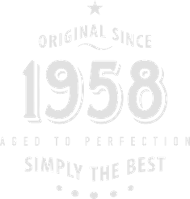3,50 €
original since 1958 simply the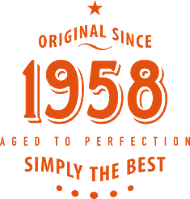3,00 €
original since 1967 simply the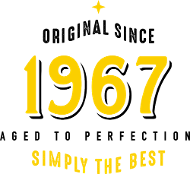3,50 €
original since 1967 simply the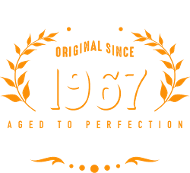3,50 €
original since 1987 simply the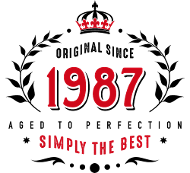3,50 €
original since 1978 simply the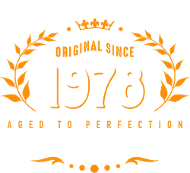3,50 €
original since 1958 simply the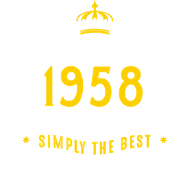3,50 €
original since 1968 simply the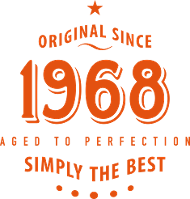3,50 €
original since 1978 simply the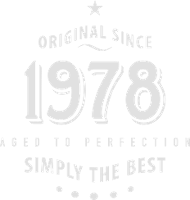3,50 €
original since 1988 simply the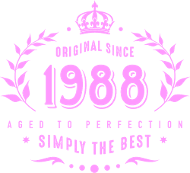3,50 €
original since 1988 simply the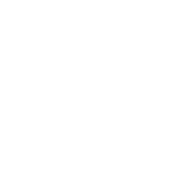3,50 €
original since 1958 simply the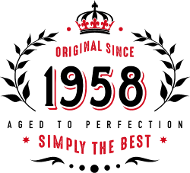3,00 €
original since 1988 simply the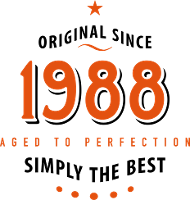3,00 €
original since 1988 simply the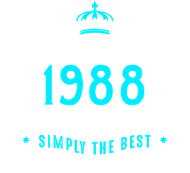3,00 €
original since 1988 simply the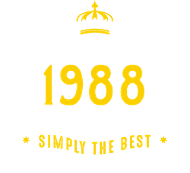3,50 €
original since 1968 simply the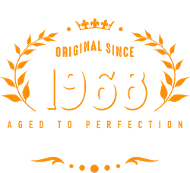3,50 €
original since 1968 simply the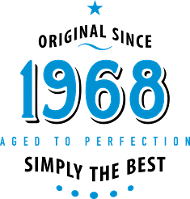3,50 €
original since 1958 simply the3,50 €
original since 1968 simply the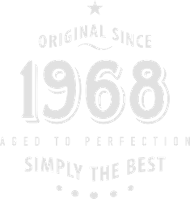3,50 €
original since 1977 simply the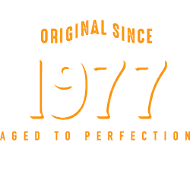3,50 €
original since 1987 simply the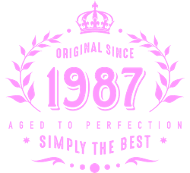3,50 €
original since 1958 simply the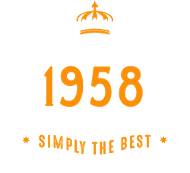3,00 €
original since 1978 simply the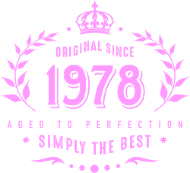3,50 €
original since 1968 simply the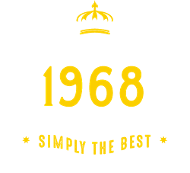3,50 €
original since 1958 simply the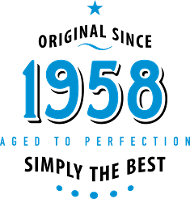3,50 €
original since 1988 simply the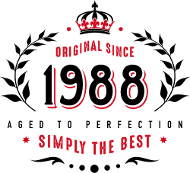3,00 €
original since 1988 simply the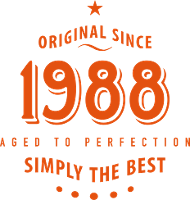3,00 €
original since 1977 simply the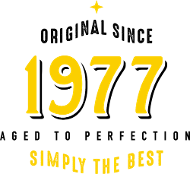3,50 €
original since 1977 simply the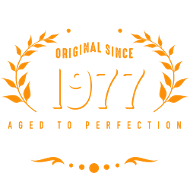3,50 €
original since 1987 simply the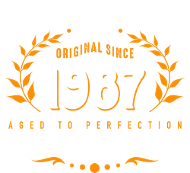3,50 €
original since 1978 simply the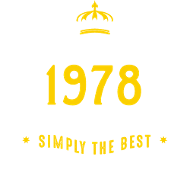3,50 €
original since 1958 simply the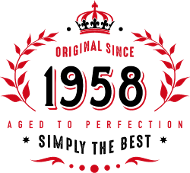3,50 €
original since 1968 simply the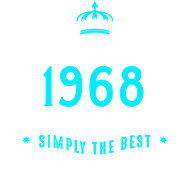3,00 €
original since 1968 simply the3,00 €
original since 1958 simply the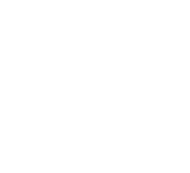3,50 €